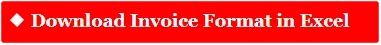=IF else( ) Formula New Trick in Excel(Advance Excel in Hindi)=IF else( ) Formula New Trick in Excel (Advance Excel in Hindi)

Watch This Video to Learn1 thought on “=IF else( ) Formula New Trick in Excel(Advance Excel in Hindi)”

1.